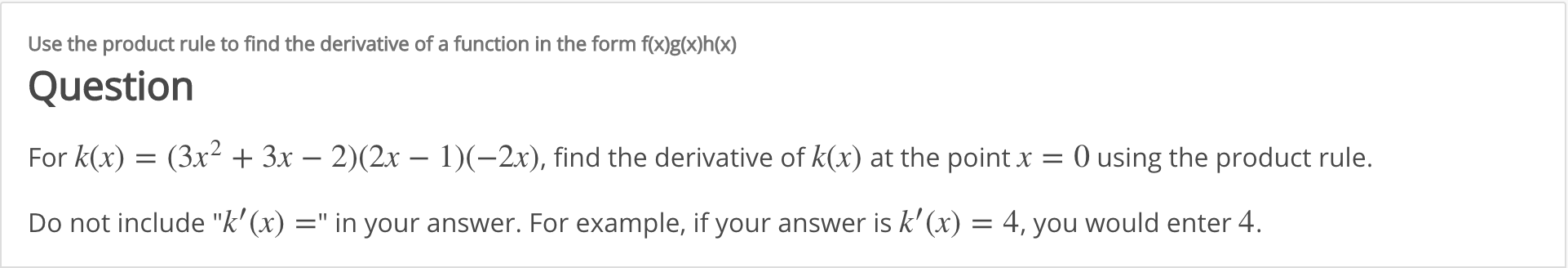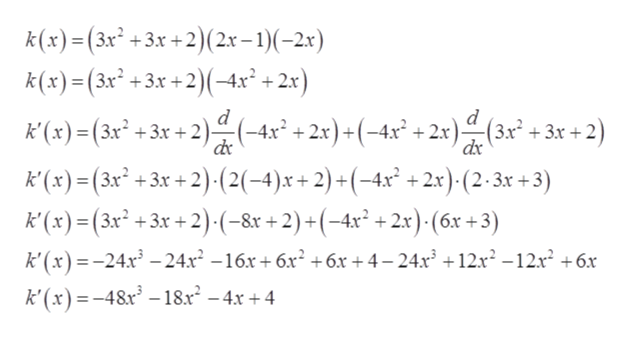# Use the product rule to find the derivative of a function in the form f(x)g(x)h(x)QuestionFor k(x) = (3x² + 3x – 2)(2x – 1)(-2x), find the derivative of k(x) at the point x = 0 using the product rule.Do not include "k' (x) =" in your answer. For example, if your answer is k'(x) = 4, you would enter 4.

Question
14 viewshelp_outlineImage TranscriptioncloseUse the product rule to find the derivative of a function in the form f(x)g(x)h(x) Question For k(x) = (3x² + 3x – 2)(2x – 1)(-2x), find the derivative of k(x) at the point x = 0 using the product rule. Do not include "k' (x) =" in your answer. For example, if your answer is k'(x) = 4, you would enter 4. fullscreen
check_circle

Step 1

Given:

Step 2

Concept used:

Step 3

Differentiate k (x) with respect ‘x&r...help_outlineImage Transcriptionclose:+2)(2x – 1)(-2x) k (x)=(3x² +3x +2)(-4x² + 2x) k(x)=(3x² +3x +2 k'(x) =(3x² +3x + 2)(-4x° + 2x)+(-4x° + 2x)(3x* + 3x k'(x)=(3x² +3x + 2)·-(2(-4)x+ 2) +(-4x² + 2.x) (2·3x +3) k'(x) = (3x² + 3x + 2)•(-&r + 2) +(-4x² + 2x) (6xr + 3) k' (x) =-24x² – 24x² –16x+ 6x² +6x +4– 24x +12.x² -12x +6x de k' (x) =-48x² – 18.r – 4x + 4 fullscreen

### Want to see the full answer?

See Solution

#### Want to see this answer and more?

Solutions are written by subject experts who are available 24/7. Questions are typically answered within 1 hour.*

See Solution
*Response times may vary by subject and question.
Tagged in

### Other